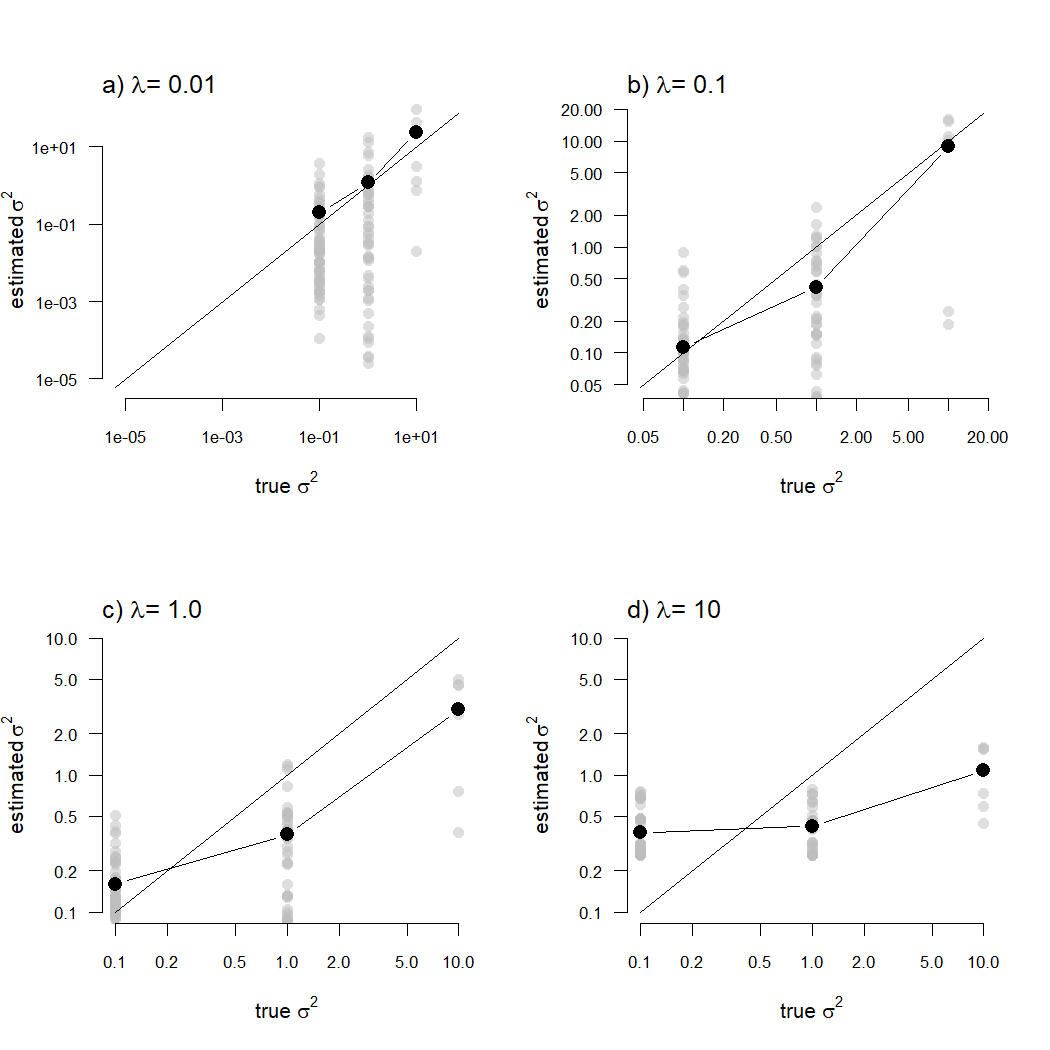## Monday, April 12, 2021

### More updates to multi-rate Brownian evolution model with examples from simulated data

Recently I've been blogging a bit about a new penalized likelihood method to fit a multi-rate Brownian evolution model to quantitative trait data & a phylogeny in which the log of the rate of evolution (σ2) itself evolves by Brownian motion (e.g., 1, 2, 3).

The way that this model works is by penalizing the likelihood in proportion to the negative of the probability of the rates under the model, multiplied by a scaling or smoothing coefficient, λ, that needs to be set by the user. For relatively high values of λ, rate variation is penalized and we eventually (for higher & higher λ) should converge to a single-rate model. For relatively low values of λ, rate variation has no cost at all and we should eventually converge on a model in which the rates are completely free to vary from edge to edge on the phylogeny just as much as might be indicated in the data.

For fun, I thought I'd simulate three different data vectors – x, y, & z – in which x evolves under a Brownian rate variation model, y under an uncorrelated rates model, and z under a rate-shift model in which there are three distinct rates in different parts of the tree. Then I'll fit our variable-rates model to each dataset for different values of λ, and compare the results.

One change I made to the current version of the model-fitting function, `multirateBM` was to add (and default to) the optimization method `"L-BFGS-B"` in `optim`. This is a Quasi-Newton optimization method with box constraints. Actually, since we are optimizing the σ2 of each branch of the tree on a log scale, we technically don't need to use a constrained optimization (any value of log(σ2), from -∞ to ∞ is theoretically allowable) – it just turns out to work better if we do!

Here we go.

``````library(phytools)
tree<-pbtree(n=60)
sig2x<-exp(fastBM(tree,internal=TRUE))
tt<-tree
tt\$edge.length<-tt\$edge.length*apply(tt\$edge,1,
function(e,x) mean(x[e]),x=sig2x)
x<-fastBM(tt)
``````

Let's create a plot with our simulated rates and data.

Here what I'll do is create a `"multirateBM"` model object, then a `"multirateBM_plot"` object (using `plot.mulirateBM` but with the argument `plot=FALSE`), then take the tree from this object & graph a traitgram using the `phenogram` function in phytools.

``````object<-list()
class(object)<-"multirateBM"
object\$sig2<-sig2x
object\$tree<-tree
object<-plot(object,plot=FALSE)
par(fg="white",bg="black",col.lab="white",
col.axis="white")
phenogram(object\$tree,x,colors=object\$cols,
``````
``````## Optimizing the positions of the tip labels...
``````
``````add.color.bar(1.25,cols=object\$cols,
title=expression(paste("log(",sigma^2,")")),
lims=range(log(sig2x)),prompt=FALSE,
x=0.25,y=5.5,subtitle="")
``````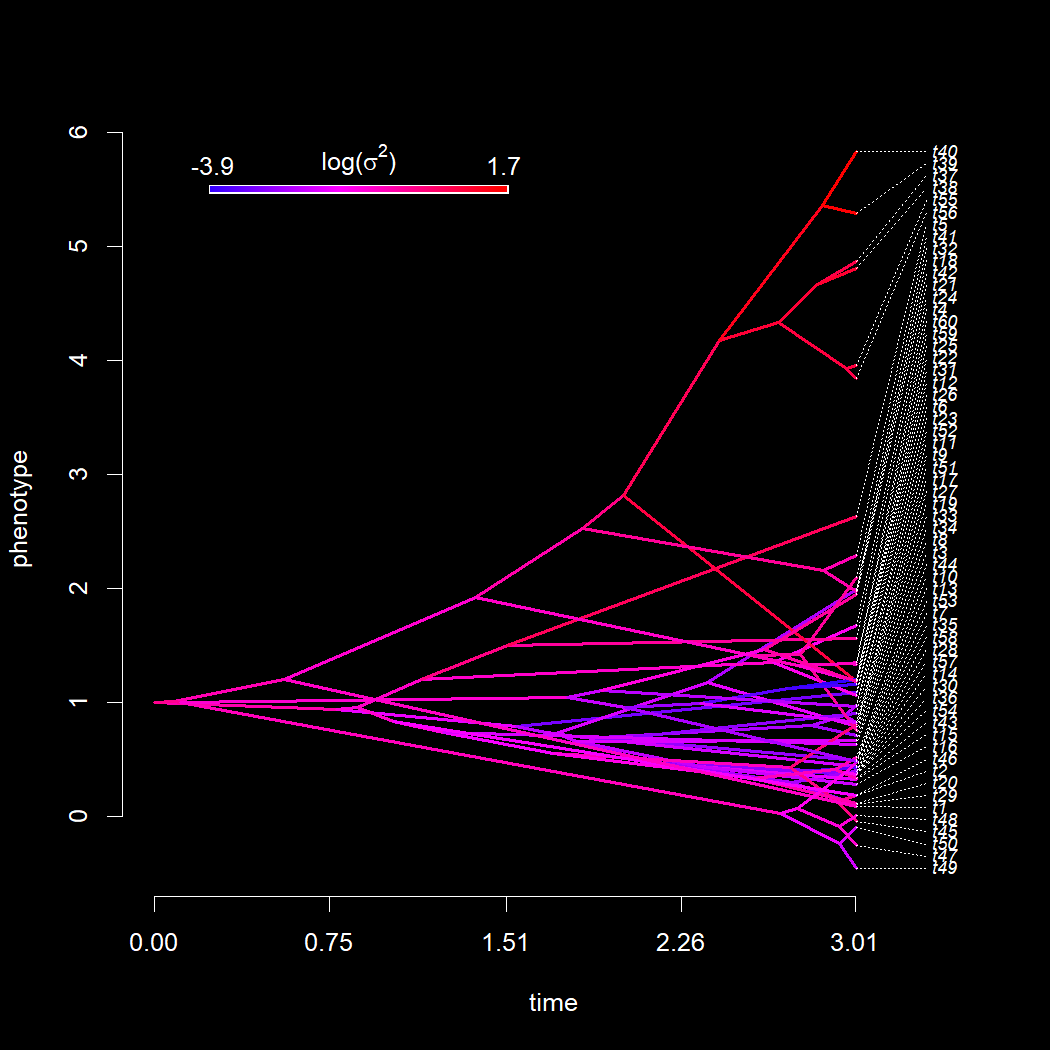Neat, it should be pretty clear that our trait, x, changes more on edges of the tree with higher (redder in the plot) evolutionary rates than along edges with lower (bluer) rates.

Next, let's use our penalized likelihood method to fit a variable-rate evolutionary model to our data and tree for different values of λ. I'm using four different values of λ, 0.01, 0.1, 1.0, and 10, but these are arbitrary.

``````lambda<-c(0.01,0.1,1.0,10.0)
fits.x<-list()
for(i in 1:length(lambda))
fits.x[[i]]<-multirateBM(tree,x,lambda=lambda[i])
``````
``````## Beginning optimization....
## Optimization iteration 1. Using "L-BFGS-B" optimization method.
## Best (penalized) log-likelihood so far: -6.39521
## Optimization iteration 2. Using "Nelder-Mead" optimization method.
## Best (penalized) log-likelihood so far: -6.26306
## Optimization iteration 3. Using "BFGS" optimization method.
## Best (penalized) log-likelihood so far: -6.06925
## Done optimization.
## Beginning optimization....
## Optimization iteration 1. Using "L-BFGS-B" optimization method.
## Best (penalized) log-likelihood so far: -25.899
## Optimization iteration 2. Using "Nelder-Mead" optimization method.
## Best (penalized) log-likelihood so far: -25.899
## Optimization iteration 3. Using "BFGS" optimization method.
## Best (penalized) log-likelihood so far: -25.8988
## Done optimization.
## Beginning optimization....
## Optimization iteration 1. Using "L-BFGS-B" optimization method.
## Best (penalized) log-likelihood so far: -65.8362
## Optimization iteration 2. Using "Nelder-Mead" optimization method.
## Best (penalized) log-likelihood so far: -65.8175
## Optimization iteration 3. Using "BFGS" optimization method.
## Best (penalized) log-likelihood so far: -65.3318
## Done optimization.
## Beginning optimization....
## Optimization iteration 1. Using "L-BFGS-B" optimization method.
## Best (penalized) log-likelihood so far: -318.392
## Optimization iteration 2. Using "Nelder-Mead" optimization method.
## Best (penalized) log-likelihood so far: -318.392
## Optimization iteration 3. Using "BFGS" optimization method.
## Best (penalized) log-likelihood so far: -316.545
## Optimization iteration 4. Using "CG" optimization method.
## Best (penalized) log-likelihood so far: -316.544
## Optimization iteration 5. Using "L-BFGS-B" optimization method.
## Best (penalized) log-likelihood so far: -316.544
## Done optimization.
``````

Now with each of these four different fitted models in hand, let's compare them back to our known simulated rates.

``````par(mfrow=c(2,2))
for(i in 1:length(fits.x)){
xylim<-range(c(sig2x,fits.x[[i]]\$sig2))
plot(sig2x,fits.x[[i]]\$sig2,log="xy",xlim=xylim,,
ylim=xylim,bty="n",las=1,pch=21,cex=1.2,
bg="grey",xlab=expression(paste("true ",sigma^2)),
ylab=expression(paste("estimated ",sigma^2)),
cex.axis=0.8)
lines(xylim,xylim)
}
``````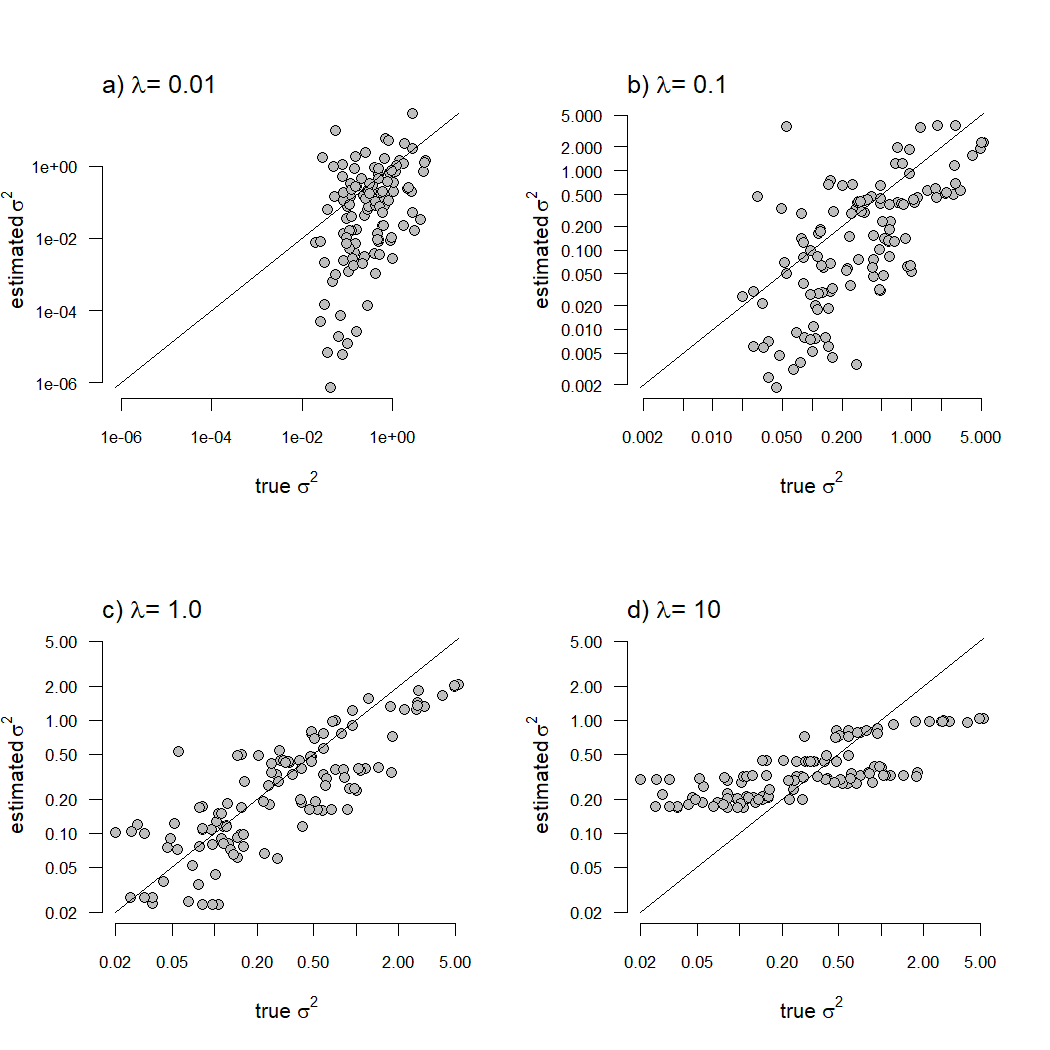Great! The effect of setting different values of the λ penalty term is pretty clear here. For greater values of λ, rate variation is penalized more and we see relatively little variation in rate among the edges of the tree. By contrast, for very small λ rate variation is penalized too little and the estimated rates vary even more than the simulated rates.

Next, let's consider the scenario of rate variation that is random across all the edges of the phylogeny.

To simulate this, I'll just pick values of log(σ2) from a normal distribution.

``````sig2y<-exp(rnorm(n=Ntip(tree)+tree\$Nnode))
tt<-tree
tt\$edge.length<-tt\$edge.length*apply(tt\$edge,1,
function(e,x) mean(x[e]),x=sig2y)
y<-fastBM(tt)
``````

If we graph this on a traitgram as we did before this is what shows up:

``````object<-list()
class(object)<-"multirateBM"
object\$sig2<-sig2y
object\$tree<-tree
object<-plot(object,plot=FALSE)
par(fg="white",bg="black",col.lab="white",
col.axis="white")
phenogram(object\$tree,y,colors=object\$cols,
``````
``````## Optimizing the positions of the tip labels...
``````
``````add.color.bar(1.25,cols=object\$cols,
title=expression(paste("log(",sigma^2,")")),
lims=range(log(sig2x)),prompt=FALSE,
x=0.25,y=5.5,subtitle="")
``````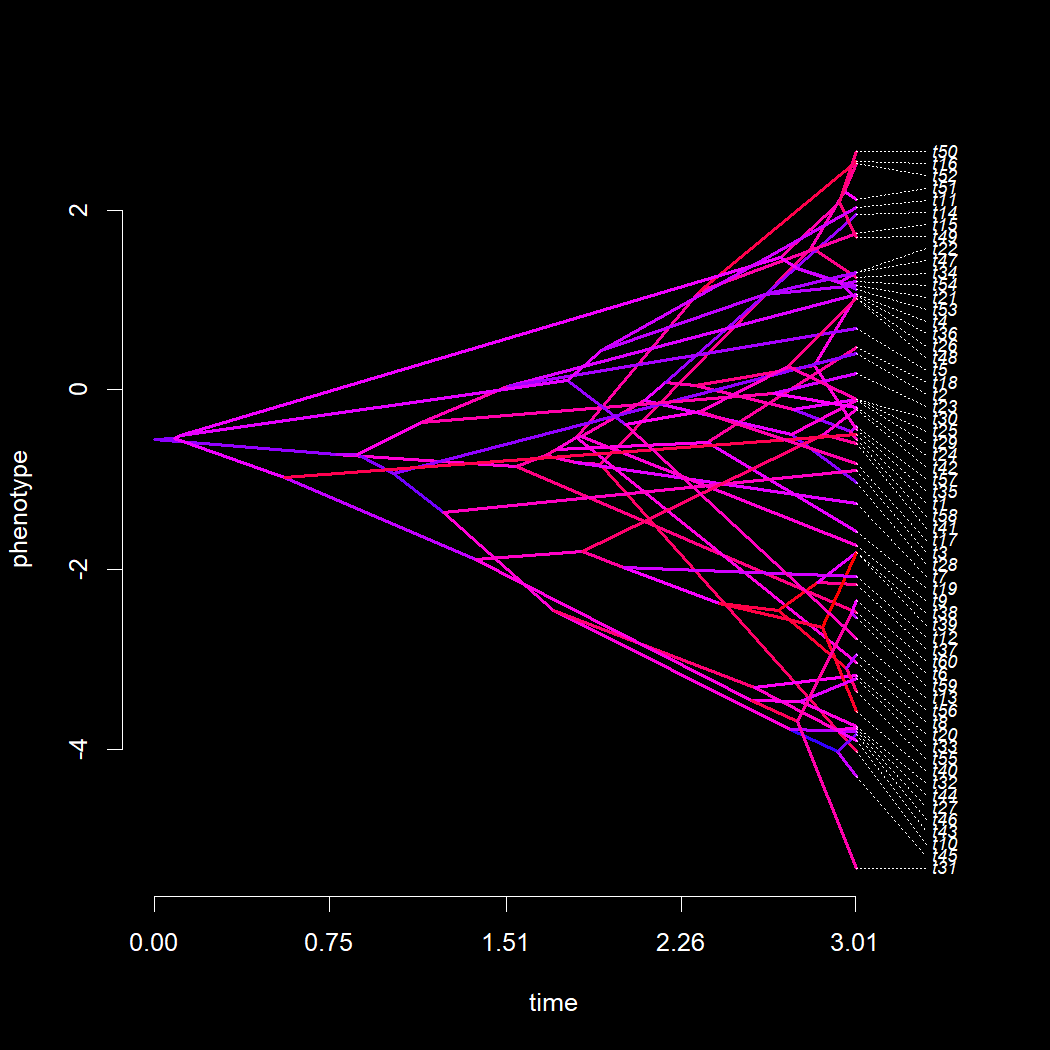Now let's fit our variable rate model using penalized likelihood, once again using the same four different values for λ that we used for x.

``````lambda<-c(0.01,0.1,1.0,10.0)
fits.y<-list()
for(i in 1:length(lambda))
fits.y[[i]]<-multirateBM(tree,y,lambda=lambda[i])
``````
``````## Beginning optimization....
## Optimization iteration 1. Using "L-BFGS-B" optimization method.
## Best (penalized) log-likelihood so far: -71.834
## Optimization iteration 2. Using "Nelder-Mead" optimization method.
## Best (penalized) log-likelihood so far: -71.8178
## Optimization iteration 3. Using "BFGS" optimization method.
## Best (penalized) log-likelihood so far: -71.7971
## Done optimization.
## Beginning optimization....
## Optimization iteration 1. Using "L-BFGS-B" optimization method.
## Best (penalized) log-likelihood so far: -87.6382
## Optimization iteration 2. Using "Nelder-Mead" optimization method.
## Best (penalized) log-likelihood so far: -87.6382
## Optimization iteration 3. Using "BFGS" optimization method.
## Best (penalized) log-likelihood so far: -87.638
## Done optimization.
## Beginning optimization....
## Optimization iteration 1. Using "L-BFGS-B" optimization method.
## Best (penalized) log-likelihood so far: -122.146
## Optimization iteration 2. Using "Nelder-Mead" optimization method.
## Best (penalized) log-likelihood so far: -122.146
## Optimization iteration 3. Using "BFGS" optimization method.
## Best (penalized) log-likelihood so far: -122.135
## Done optimization.
## Beginning optimization....
## Optimization iteration 1. Using "L-BFGS-B" optimization method.
## Best (penalized) log-likelihood so far: -366.965
## Optimization iteration 2. Using "Nelder-Mead" optimization method.
## Best (penalized) log-likelihood so far: -366.965
## Optimization iteration 3. Using "BFGS" optimization method.
## Best (penalized) log-likelihood so far: -366.883
## Optimization iteration 4. Using "CG" optimization method.
## Best (penalized) log-likelihood so far: -366.882
## Optimization iteration 5. Using "L-BFGS-B" optimization method.
## Best (penalized) log-likelihood so far: -366.882
## Done optimization.
``````

As we did for x, why don't we compare the estimated values of σ2 under our model, to the simulated values of σ2, for each of our different penalty term coefficient (λ) values.

``````par(mfrow=c(2,2))
for(i in 1:length(fits.y)){
xylim<-range(c(sig2y,fits.y[[i]]\$sig2))
plot(sig2y,fits.y[[i]]\$sig2,log="xy",xlim=xylim,,
ylim=xylim,bty="n",las=1,pch=21,cex=1.2,
bg="grey",xlab=expression(paste("true ",sigma^2)),
ylab=expression(paste("estimated ",sigma^2)),
cex.axis=0.8)
lines(xylim,xylim)
}
``````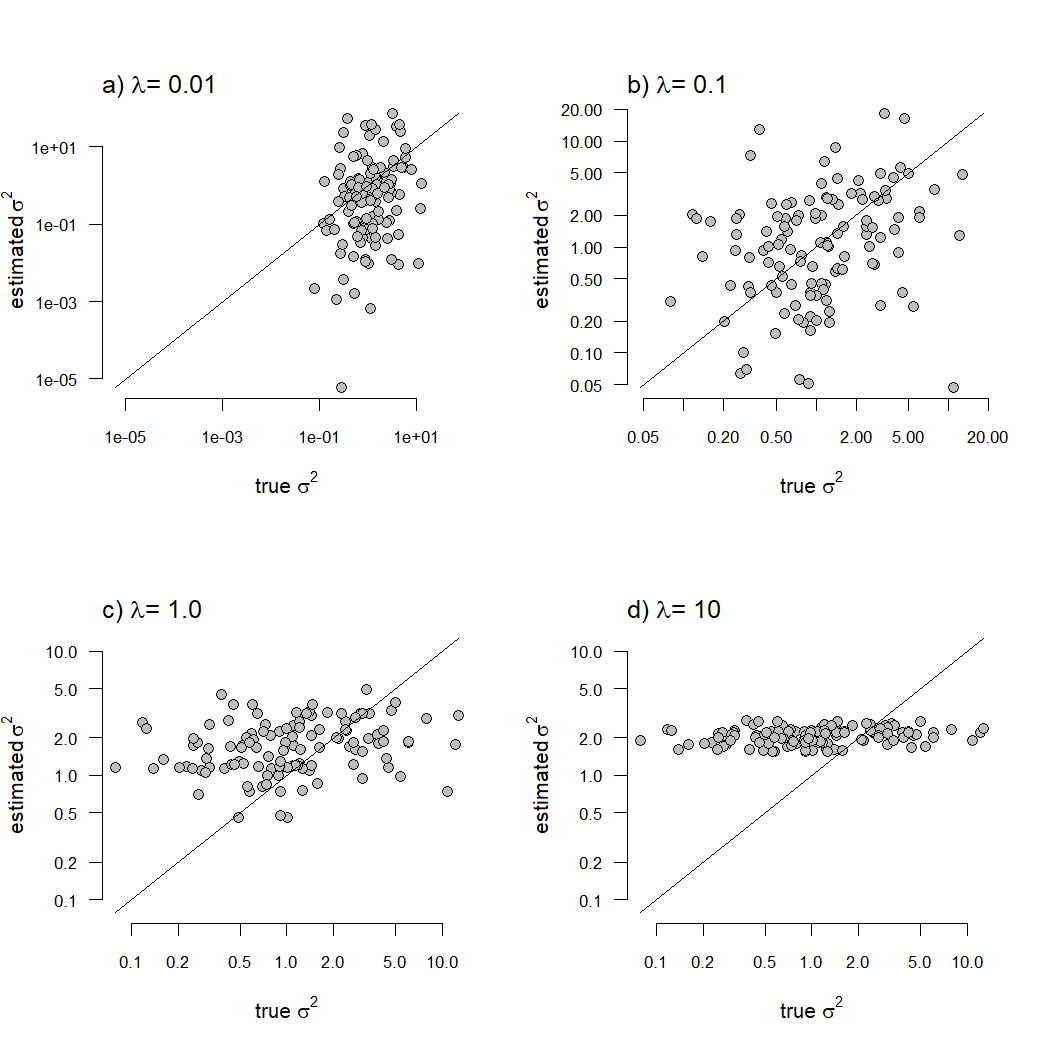In this case, we see a stronger correlation between our known & estimated rates for lower values of λ than we did for x. Furthermore, this correlation is weaker overall than when σ2 evolved by Brownian motion.

Lastly, let's imagine a discrete rate shift on the phylogeny in which all the edges on one side of the shift evolved with one rate, and all the edges on the other side evolved with another. In this case, I'll simulate three different regimes which will correspond to three different rates: σ2 = 0.1, 1.0, and 10.

To do this I'll first evolve a discrete character trait with three levels, then I'll “fix” all the edges of the tree with state changes so that the change occurs precisely at the midpoint of the edge. (The reason for this is because in our penalized likelihood model all nodes and tips of the tree have values for σ2, and the model assumes Brownian evolution on a log-scale between the values at each nodes. This means that the expected value for log(σ2) at the midpoint of an edge is precisely intermediate between the two endpoints.) I'll also only allow transitions to occur between adjacent values of σ2 (that is from 0.1 to 1.0 and vice versa, or from 1.0 to 10 and vice versa, but not from 0.1 to 10), and I'll screen my simulations to include only trees in which no greater than one change occurred on each edge. (I did this for simplicity in analyzing our results.)

``````Q<-matrix(c(-0.2,0.2,0,
0.2,-0.4,0.2,
0,0.2,-0.2),3,3,
byrow=TRUE,
dimnames=list(1:3,1:3))
map<-sim.history(tree,Q,anc="2",quiet=TRUE)
``````
``````## Done simulation(s).
``````
``````while(any(sapply(map\$maps,length)>2))
map<-sim.history(tree,Q,anc="2",quiet=TRUE)
``````
``````## Done simulation(s).
## Done simulation(s).
## Done simulation(s).
## Done simulation(s).
## Done simulation(s).
## Done simulation(s).
## Done simulation(s).
## Done simulation(s).
## Done simulation(s).
``````
``````for(i in 1:length(map\$maps)){
if(length(map\$maps[[i]])>1){
map\$maps[[i]]<-setNames(rep(map\$edge.length[i]/2,2),
names(map\$maps[[i]]))
}
}
``````
``````## map order should be specified in function call or by tree attribute "map.order".
## Assuming right-to-left order.
``````
``````z<-sim.rates(map,sig2=setNames(c(0.1,1,10),1:3))
``````

Let's plot the result of our simulation just as we did for x and y.

``````par(fg="white",bg="black",col.lab="white",
col.axis="white")
cols<-setNames(colorRampPalette(c("blue","red"))(3),1:3)
phenogram(map,z,colors=cols,
``````
``````## Optimizing the positions of the tip labels...
``````
``````legend("topleft",legend=c(
expression(paste(sigma^2," = 0.1")),
expression(paste(sigma^2," = 1.0")),
expression(paste(sigma^2," = 10"))),
pch=22,pt.bg=cols,pt.cex=2,bty="n")
``````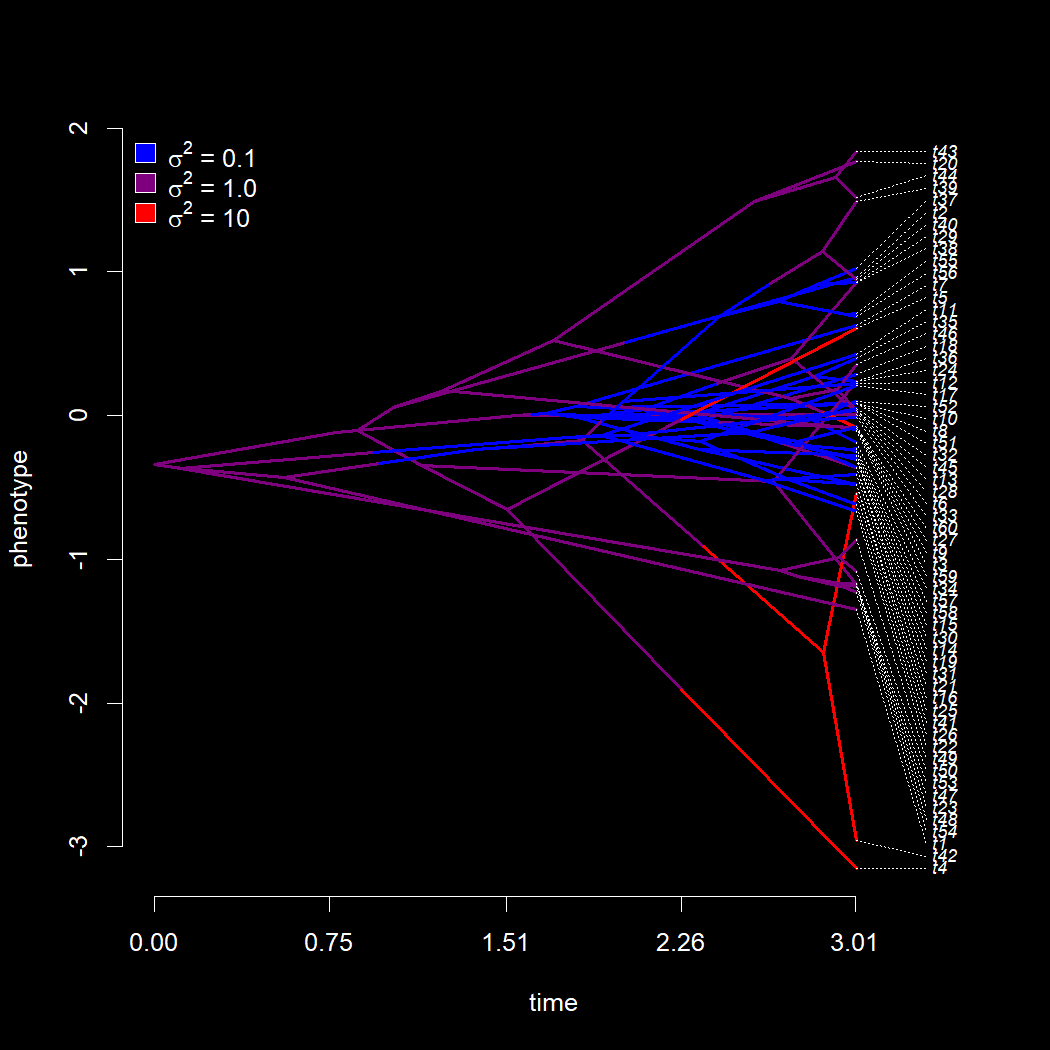Finally, once again, let's fit our different models using `multirateBM` and then plot the simulated rates against the estimated rates under each of the four different λ values that we used.

``````sig2z<-c(getStates(map,"tips"),getStates(map,"nodes"))
sig2z<-setNames(setNames(c(0.1,1,10),1:3)[sig2z],names(sig2z))
lambda<-c(0.01,0.1,1.0,10.0)
fits.z<-list()
for(i in 1:length(lambda))
fits.z[[i]]<-multirateBM(tree,z,lambda=lambda[i])
``````
``````## Beginning optimization....
## Optimization iteration 1. Using "L-BFGS-B" optimization method.
## Best (penalized) log-likelihood so far: -2.86288
## Optimization iteration 2. Using "Nelder-Mead" optimization method.
## Best (penalized) log-likelihood so far: -2.82511
## Optimization iteration 3. Using "BFGS" optimization method.
## Best (penalized) log-likelihood so far: -2.79261
## Done optimization.
## Beginning optimization....
## Optimization iteration 1. Using "L-BFGS-B" optimization method.
## Best (penalized) log-likelihood so far: -25.2168
## Optimization iteration 2. Using "Nelder-Mead" optimization method.
## Best (penalized) log-likelihood so far: -25.2168
## Optimization iteration 3. Using "BFGS" optimization method.
## Best (penalized) log-likelihood so far: -25.216
## Done optimization.
## Beginning optimization....
## Optimization iteration 1. Using "L-BFGS-B" optimization method.
## Best (penalized) log-likelihood so far: -64.962
## Optimization iteration 2. Using "Nelder-Mead" optimization method.
## Best (penalized) log-likelihood so far: -64.962
## Optimization iteration 3. Using "BFGS" optimization method.
## Best (penalized) log-likelihood so far: -64.923
## Done optimization.
## Beginning optimization....
## Optimization iteration 1. Using "L-BFGS-B" optimization method.
## Best (penalized) log-likelihood so far: -325.015
## Optimization iteration 2. Using "Nelder-Mead" optimization method.
## Best (penalized) log-likelihood so far: -324.969
## Optimization iteration 3. Using "BFGS" optimization method.
## Best (penalized) log-likelihood so far: -322.516
## Done optimization.
``````
``````par(mfrow=c(2,2))
for(i in 1:length(fits.z)){
xylim<-range(c(sig2z,fits.y[[i]]\$sig2))
plot(sig2z,fits.z[[i]]\$sig2,log="xy",xlim=xylim,,
ylim=xylim,bty="n",las=1,pch=16,cex=1.2,
col=make.transparent("grey",0.5),
xlab=expression(paste("true ",sigma^2)),
ylab=expression(paste("estimated ",sigma^2)),
cex.axis=0.8)
levs<-c(0.1,1,10)
lines(levs,sapply(levs,function(x,y,z) mean(y[z==x]),
y=fits.z[[i]]\$sig2,z=sig2z),type="b",pch=16,
cex=1.5)
lines(xylim,xylim)